Question

# A unity feedback system with the forward transfer function G)2)(s +5) is operating with a closed-...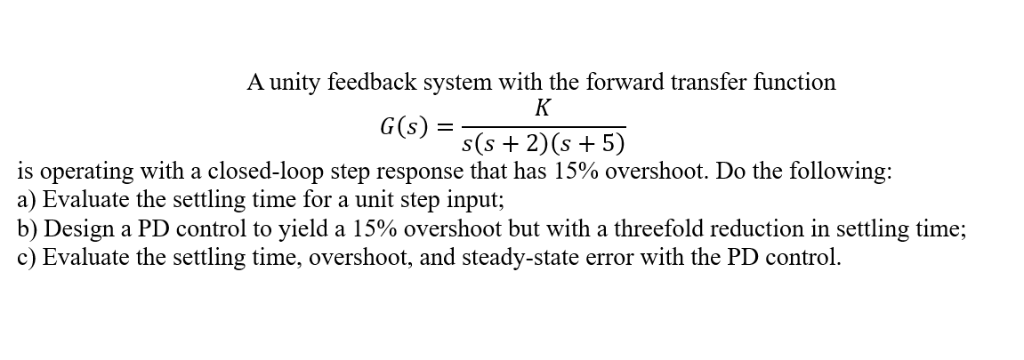A unity feedback system with the forward transfer function G)2)(s +5) is operating with a closed-loop step response that has 15% overshoot. Do the following: a) Evaluate the settling time for a unit step input; b) Design a PD control to yield a 15% overshoot but with a threefold reduction in settling time; c) Evaluate the settling time, overshoot, and steady-state error with the PD control.

I have used MATLAB to find out the gain K where the overshoot is 15%.

MATLAB code to plot the rlocus is given below.

clc;
close all;
clear all;

s = tf('s');
k = 0:0.1:20;
G = 1/(s*(s+2)*(s+5));
figure;rlocus(G,k);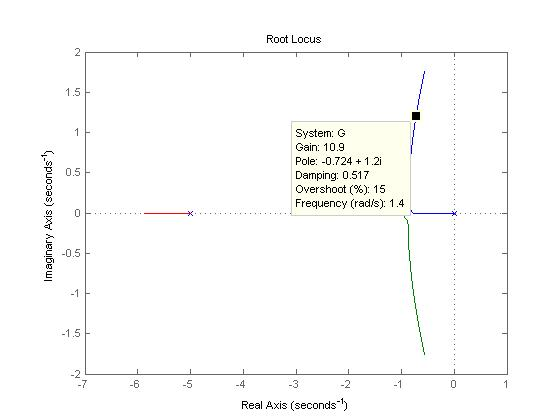From the above figure, it is observed that at gain 10.9, the overshoot is 15%.

Therefore k = 10.9.

settling time for unit step is given by Ts = 4 time constants = 4/ |real part of dominant pole = 4/ 0.724 = 5.52 sec

Now it is required to design a PD controller to meet 15% overshoot but settling time = 5.52/3 = 1.84 sec.

I have used root locus method to determine the PD controller.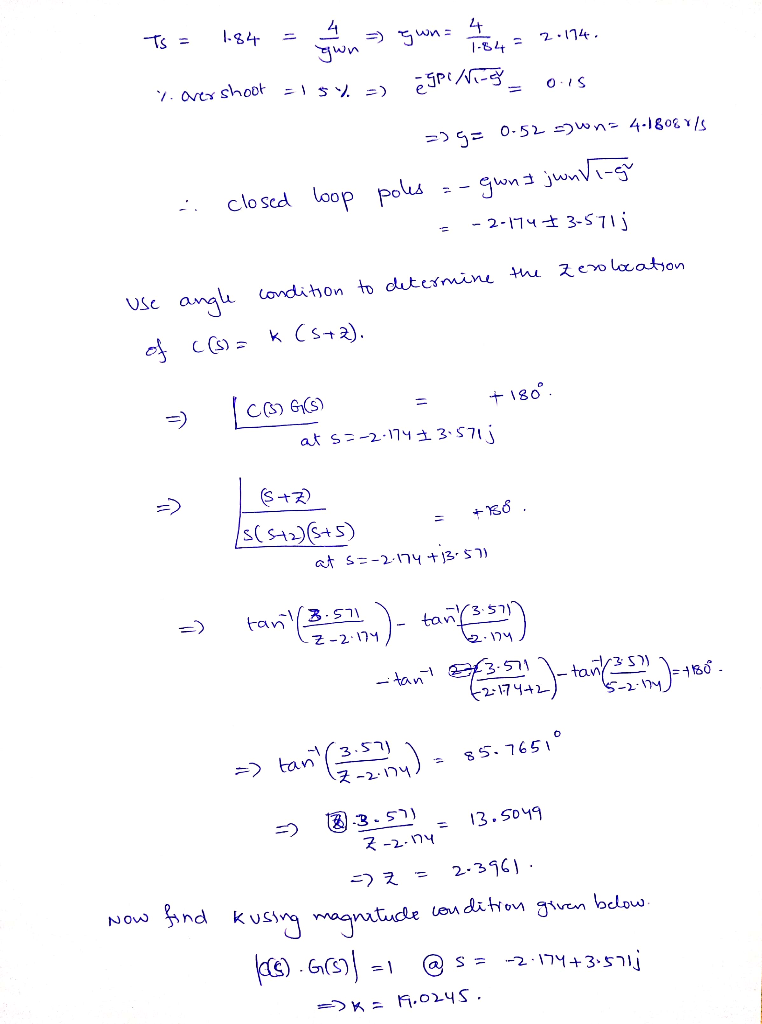Therefore the controller is given by C(s) = 19.0245(s+2.3961)

I have used MATLAB to plot he response with the controller.

code:

C = 19.0245*(s+2.3961);
figure;step(feedback(C*G,1));

Response is plotted below.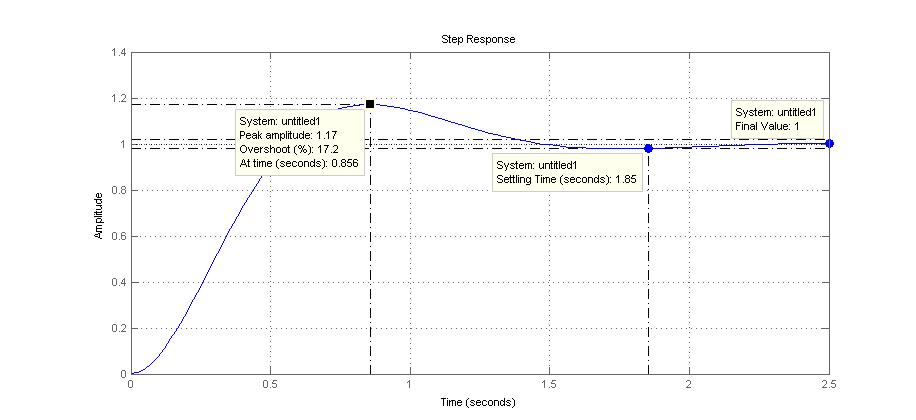From the above response it is observed that the settling time is 1.84 sec. steady state error is zero and peak overshoot is 17%

#### Earn Coins

Coins can be redeemed for fabulous gifts.

Similar Homework Help Questions
• ### A unity feedback system with the forward transfer function G (s) = s(s+2)(s15) is operating with ...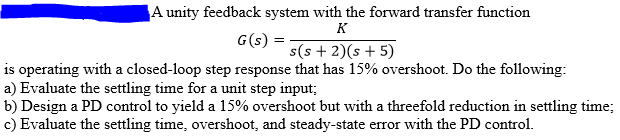A unity feedback system with the forward transfer function G (s) = s(s+2)(s15) is operating with a closed-loop step response that has 15% overshoot. Do the following: a) Evaluate the settling time for a unit step input b) Design a PD control to yield a 15% overshoot but with a threefold reduction in settling time; c) Evaluate the settling time, overshoot, and steady-state error with the PD control. A unity feedback system with the forward transfer function G (s) =...

• ### A unity feedback system with the forward transfer function G(s)=K/(s+1)(s+3)(s+6) is operating wi...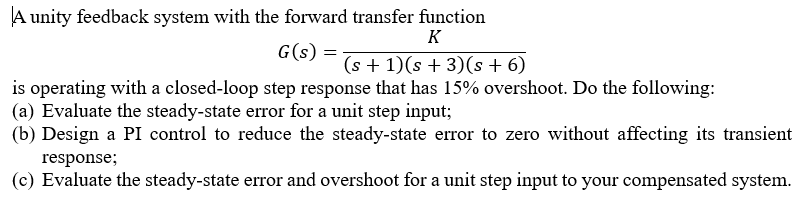A unity feedback system with the forward transfer function G(s)=K/(s+1)(s+3)(s+6) is operating with a closed-loop step response that has 15% overshoot. Do the following: a) Evaluate the steady-state error for a unit step input b) Design a PI control to reduce the steady-state error to zero without affecting its transient response c) Evaluate the steady-state error and overshoot for a unit step input to your compensated system A unity feedback system with the forward transfer function G(s) is operating with...

• ### Problem 1. A unity feedback system with forward transfer function G(s) is operating with a closed...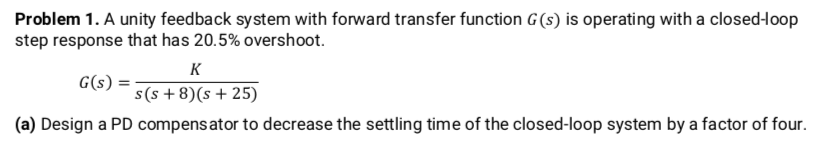Problem 1. A unity feedback system with forward transfer function G(s) is operating with a closed-loop step response that has 20.5% overshoot. G)-(+8)6 + 25) G(s) (a) Design a PD compens ator to decrease the settling time of the closed-loop system by a factor of four Problem 1. A unity feedback system with forward transfer function G(s) is operating with a closed-loop step response that has 20.5% overshoot. G)-(+8)6 + 25) G(s) (a) Design a PD compens ator to decrease...

• ### PROBLEM: A unity feedback system with the forward transfer function K G(s) s(s+7) is operating with...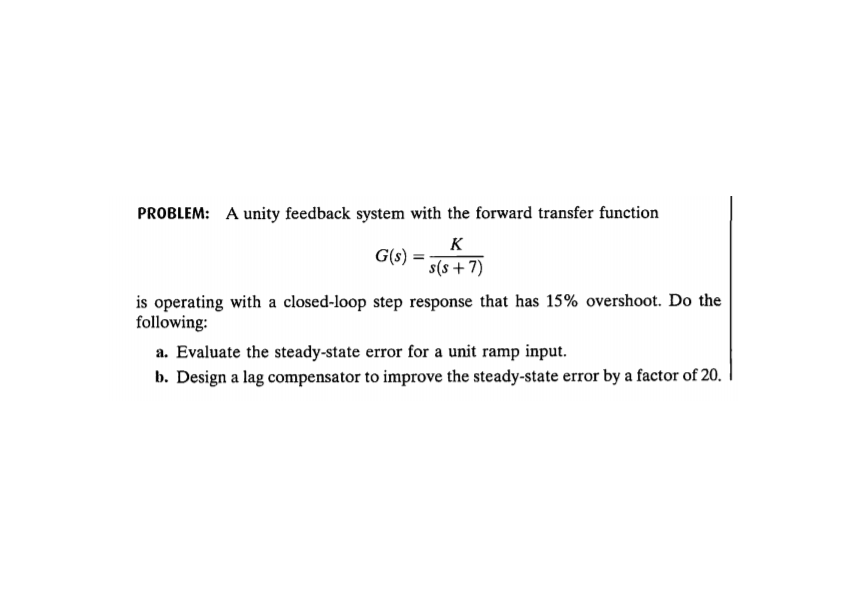PROBLEM: A unity feedback system with the forward transfer function K G(s) s(s+7) is operating with a closed-loop step response that has 15% overshoot. Do the following: a. Evaluate the steady-state error for a unit ramp input. b. Design a lag compensator to improve the steady-state error by a factor of 20. c. Evaluate the steady-state error for a unit ramp input to your compensated system. d. Evaluate how much improvement in steady-state error was realized.

• ### 1. Consider a unity feedback control system with the transfer function G(s) = 1/[s(s+ 2)] in...

1. Consider a unity feedback control system with the transfer function G(s) = 1/[s(s+ 2)] in the forward path. (a) Design a proportional controller that yields a stable system with percent overshoot less that 5% for the step input (b) Find settling time and peak time of the closed-loop system designed in part (a); (c) Design a PD compensator that reduces the settling time computed in (b) by a factor of 4 while keeping the percent overshoot less that 5%...

• ### Please show calculations by HAND and NOT MATLAB. The answers are here to help. Thank you Note : Ts= 4/&*wn (&=damping ratio) Skill-Assessment Exercise 9.3 PROBLEM: A unity feedback system wit...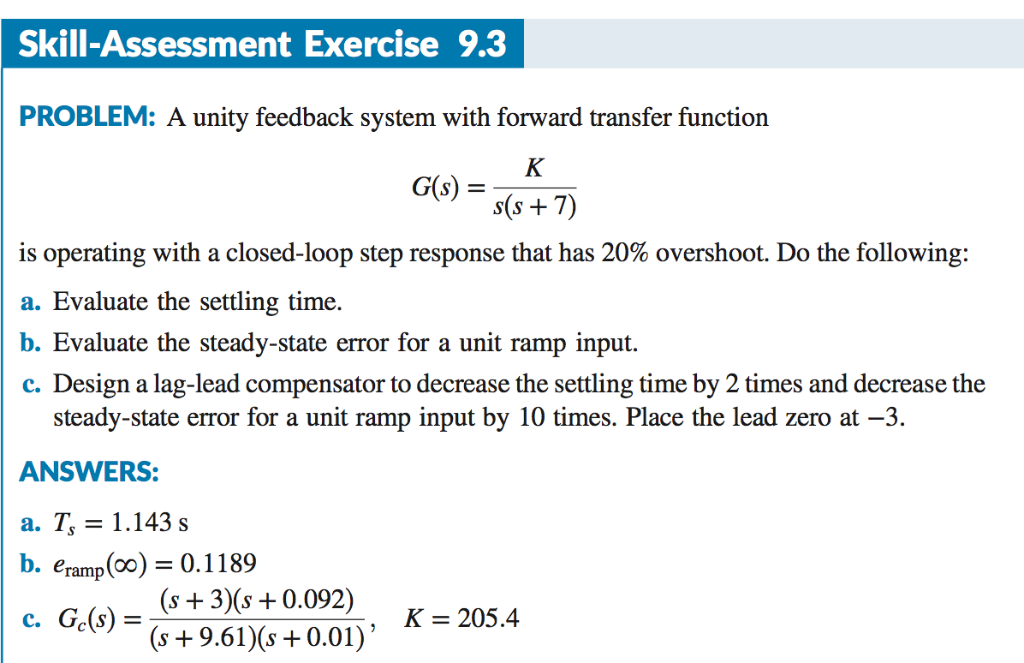Please show calculations by HAND and NOT MATLAB. The answers are here to help. Thank you Note : Ts= 4/&*wn (&=damping ratio) Skill-Assessment Exercise 9.3 PROBLEM: A unity feedback system with forward transfer function 6) s(s + is operating with a closed-loop step response that has 20% overshoot. Do the following: a. Evaluate the settling time. b. Evaluate the steady-state error for a unit ramp input. c. Design a lag-lead compensator to decrease the settling time by 2 times and...

• ### SS10. The unity-feedback system of Figure P11.1 with K (s +4) G (s) (s 2) (s 5) (s +12) is operat...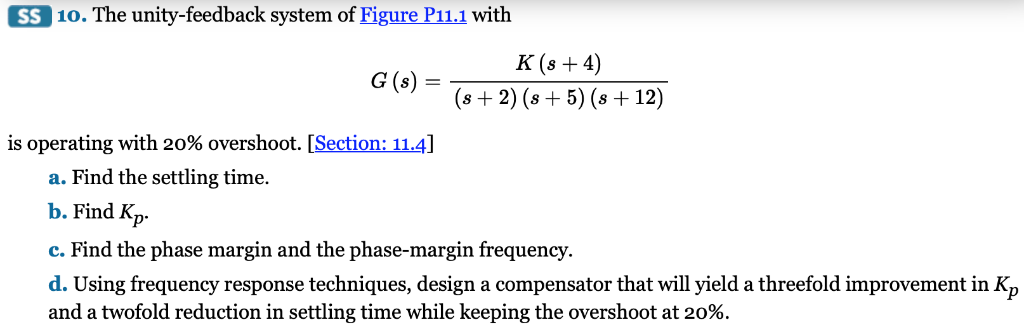SS10. The unity-feedback system of Figure P11.1 with K (s +4) G (s) (s 2) (s 5) (s +12) is operating with 20% overshoot. [Section: 114] a. Find the settling time. b. Find Kp c. Find the phase margin and the phase-margin frequency d. Using frequency response techniques, design a compensator that will yield a threefold improvement in Kp and a twofold reduction in settling time while keeping the overshoot at 20%. SS10. The unity-feedback system of Figure P11.1 with...

• ### 2. You are given the motor whose transfer function is shown in Figure 2(a). s) e(s) Amplifier Mot...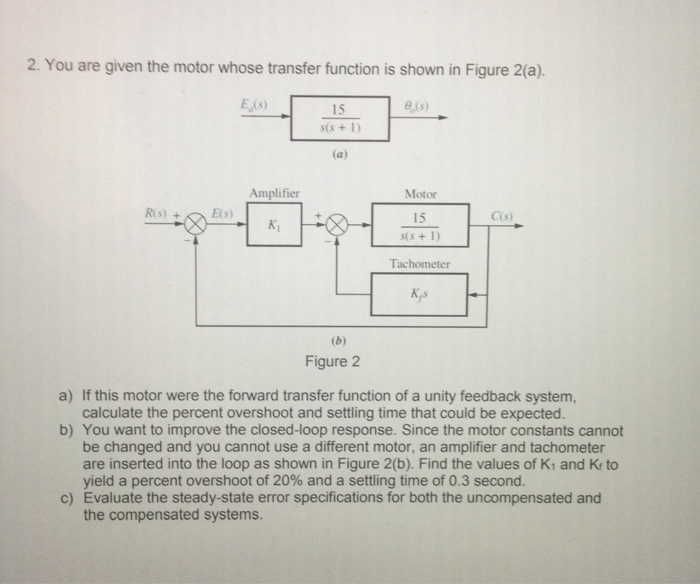Control system 2. You are given the motor whose transfer function is shown in Figure 2(a). s) e(s) Amplifier Motor C(s) 15 Tachometer Кр Figure 2 a) If this motor were the forward transfer function of a unity feedback system, calculate the percent overshoot and settling time that could be expected. b) You want to improve the closed-loop response. Since the motor constants cannot be changed and you cannot use a different motor, an amplifier and tachometer are inserted into...

• ### 1. A unity feedback system with its forward transfer function G(s) - K(s+a)/s(s+B) is to be...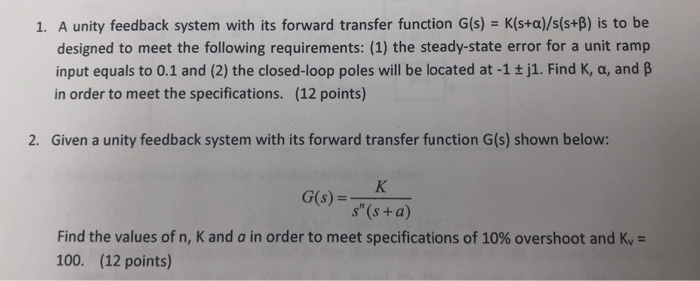1. A unity feedback system with its forward transfer function G(s) - K(s+a)/s(s+B) is to be designed to meet the following requirements: (1) the steady-state error for a unit ramp input equals to 0.1 and (2) the closed-loop poles will be located at -1 + j1. Find K, a, and B in order to meet the specifications. (12 points) 2. Given a unity feedback system with its forward transfer function G(s) shown below: s" (s +a) Find the values of...

• ### 14. The unity feedback system of Figure P1 1.1 with K(s+ 4) (s+2(s+5)(s+12) G(s) is operating...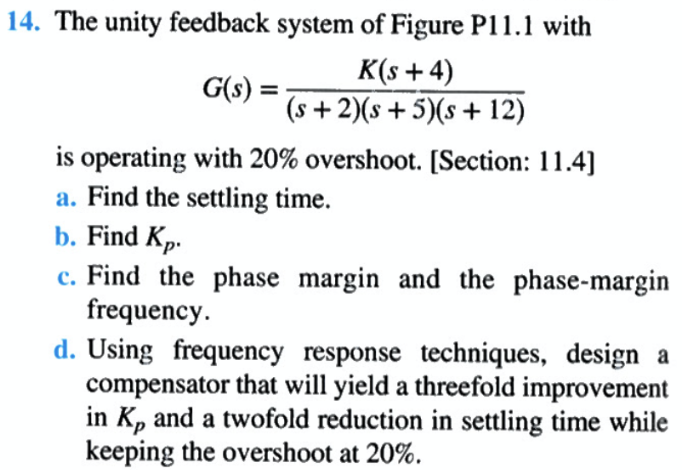14. The unity feedback system of Figure P1 1.1 with K(s+ 4) (s+2(s+5)(s+12) G(s) is operating with 20% overshoot. [Section: 11.4 a. Find the settling time b. Find Kp c. Find the phase margin and the phase-margin frequency d. Using frequency response techniques, design a compensator that will yield a threefold improvement in Kp and a twofold reduction in settling time while keeping the overshoot at 20%.